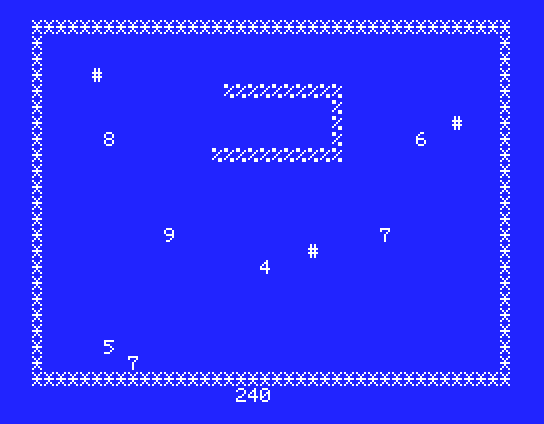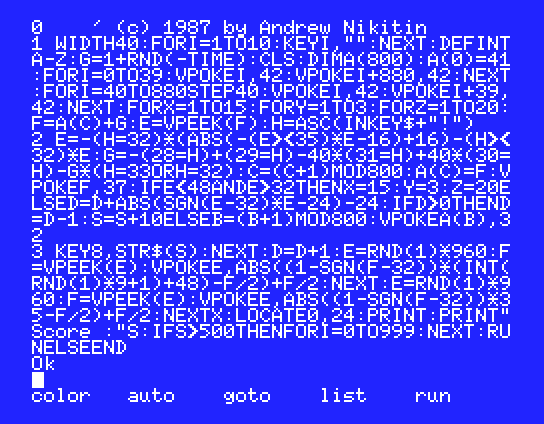#Snake

This is a remake of a popular microcomputer game SNAKE. I do not know who originally came up with the idea for the game (or, even, on what microcomputer it appeared first). The implementation I saw was 30-something lines of code, which was amazingly short for such a nice and seemingly complex game.

## RulesControl snake that crawls across the screen. Avoid walls, stones ('#') and biting own body. Eat digits that randomly appear on the screen. Each eaten digit causes snake to grow. The goal is to grow as much as you can.

There is a way to clear stones from the way: press space bar when snake's head is right before stone and stone will disappear. Timing is crucial: too early or too late and you will hit the stone, causing game over.Original 30-something line code was not terribly complicated or awfully long. It did have, however, quite a few "IF"s -- more than would comfortably fit into 3 lines. Some of those ifs seemed inevitable. How would you read keyboard input and assign new direction without at least 4 "IF"s?

Below is unfolded, spaceified and commented code.
 ```'1 WIDTH 40 FOR I=1 TO 10 KEY I,"" NEXT ``` This clears function key hints, so they are not displayed. Easier way would be to use KEY OFF operator, but I needed them on, so I could youse hint for "F8" to display current score.
 ```DEFINT A-Z G=1+RND(-TIME) CLS DIM A(800) A(0)=41 ``` Initialize G (direction) with 1 ("to the right") and also randomize random seed using timer at the same time. Since DEFINT A-Z is in action, G will remain 1.
 ```FOR I=0 TO 39 VPOKE I,42 VPOKE I+880,42 NEXT ``` Horizotal walls -- one on top, one on bottom.
 ```FOR I=40 TO 880 STEP40 VPOKE I,42 VPOKE I+39,42 NEXT ``` Vertical walls -- one on left, one on right. Walls are composed of "*" character and cannot be wiped with a space bar.
 ```FOR X=1 TO 15 FOR Y=1 TO 3 FOR Z=1 TO 20 ``` Main loop
 ``` F=A(C)+G '2 E=VPEEK(F) H=ASC(INKEY\$+"!") ```
 ``` E=-(H=32)*(ABS(-(E><35)*E-16)+16)-(H><32)*E ``` ' if h=" " and e="#" then e=" "
 ' if h=28 then g=1 else if h=29 then g=-1 else if h=30 then g=-40 else if g=31 then g=40 else if h=33 or h=32 then g=g else g=0 ``` G=-(28=H)+(29=H)-40*(31=H)+40*(30=H)-G*(H=33ORH=32) C=(C+1) MOD 800 A(C)=F VPOKE F,37 IF E<48 AND E>32 THEN ```
 ``` X=15 Y=3 Z=20 ``` Exit main loop
 ``` ELSE D=D+ABS(SGN(E-32)*E-24)-24 IF D>0 THEN D=D-1 S=S+10 ELSE B=(B+1) MOD 800 VPOKEA(B),32 [endif] [endif] '3 KEY 8,STR\$(S) NEXT D=D+1 E=RND(1)*960 F=VPEEK(E) VPOKE E,ABS((1-SGN(F-32))*(INT(RND(1)*9+1)+48)-F/2)+F/2 NEXT E=RND(1)*960 F=VPEEK(E) VPOKE E,ABS((1-SGN(F-32))*35-F/2)+F/2 NEXT X LOCATE 0,24 PRINT PRINT"Score :"S IF S>500 THEN FOR I=0 TO 999 NEXT RUN ELSE END [endif] ```
This game layed groundwork for the rest of the series. For the first time here I used comparison and logical operators in arithmetic expressions, which allowed significantly simplify program structure and reduce size. Without this tecnique most of the following would not be possible.

[ MSX BASIC | << Previous | Next >> | Feedback ]